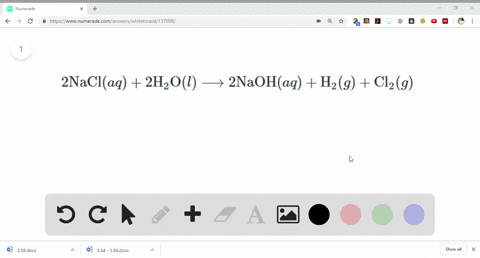Sign up for our free STEM online summer camps starting June 1st!View Summer Courses### A chemical plant uses electrical energy to decomp…

06:27University of New Mexico

Need more help? Fill out this quick form to get professional live tutoring.

Get live tutoring
Problem 99

A method used by the U.S. Environmental Protection Agency $($ EPA) for determining the concentration of ozone in airis to pass the air sample through a "bubbler" containing sodium iodide, which removes the ozone according to the following equation:
$$\begin{array}{c}{\mathrm{O}_{3}(g)+2 \operatorname{NaI}(a q)+\mathrm{H}_{2} \mathrm{O}(l) \longrightarrow} \\ \quad \quad \quad \quad \quad \quad\quad \quad \quad \quad \quad \quad \quad \quad \quad \quad \quad{\mathrm{O}_{2}(g)+\mathrm{I}_{2}(s)+2 \mathrm{NaOH}(a q)}\end{array}$$
(a) How many moles of sodium iodide are needed to remove $5.95 \times 10^{-6} \mathrm{mol}$ of $\mathrm{O}_{3} ?(\mathbf{b})$ How many grams of sodium iodide are needed to remove 1.3 $\mathrm{mg}$ of $\mathrm{O}_{3} ?$

(a) $7.6 \times 10^{-5}$ moles sodium iodide
(b) 0.00344 g NaI

## Discussion

You must be signed in to discuss.

## Video Transcript

for this question. First, we need to deter mined the the action for removal off zone. So that will be our part. Eh? Was on his old three plus two. So what he admired died lasts. It's toe oh, which gives us or two plus I to less to any which. Secondly, to calculate the moles off sodium iodide need to remove 3.8 into 10 days to pull negative five Morse off his own. So we know that one mole off a tree is removed by two moles off sodium iodide hands 3.8 into 10 days to bar five molds will be removed by to mortals off in the eye, divided by one mole off watery multiplied by 3.8 into 10 days to poor minus five, which gives us 7.6 into 10 days to bar minus fight. So 7.6 in 10 days to put negative five moles off sodium iodide will be used to remove 3.8 into 10 days to minus five miles off his own. Continuing. We need to calculate the molar mass off soon on sodium iodide so was owned or three that is three multiplied with 16 molecular weight off excision. Give this 48 on sodium iodide B 23 4 sodium plus 1 26 When 26 4 IA Dean gives us 1 26.9 Gives us 100 and 50 grams. Approximately further along, we need to calculate the mass off sodium iodide used to remove 0.55 zero grams off would or three we know that 48 gram off oxygen for it. Eight gram off ozone is removed by two in tow. 10 days to power. Let me do it again. So 48 gram off ozone is removed by two multiplied with 100 and 50 grams off sodium iodide, hence 0.550 into turned rest upon my next three Graham off. All three will be removed by as shown two multiplied with 100 and 50 divided by 48.0 multiplied with little 0.550 into 10 days. Super minus three, which gives us three point for 37 multiplied pretenders were minus three grams, so the mass off sodium require will be a 3.44 Millie rounds